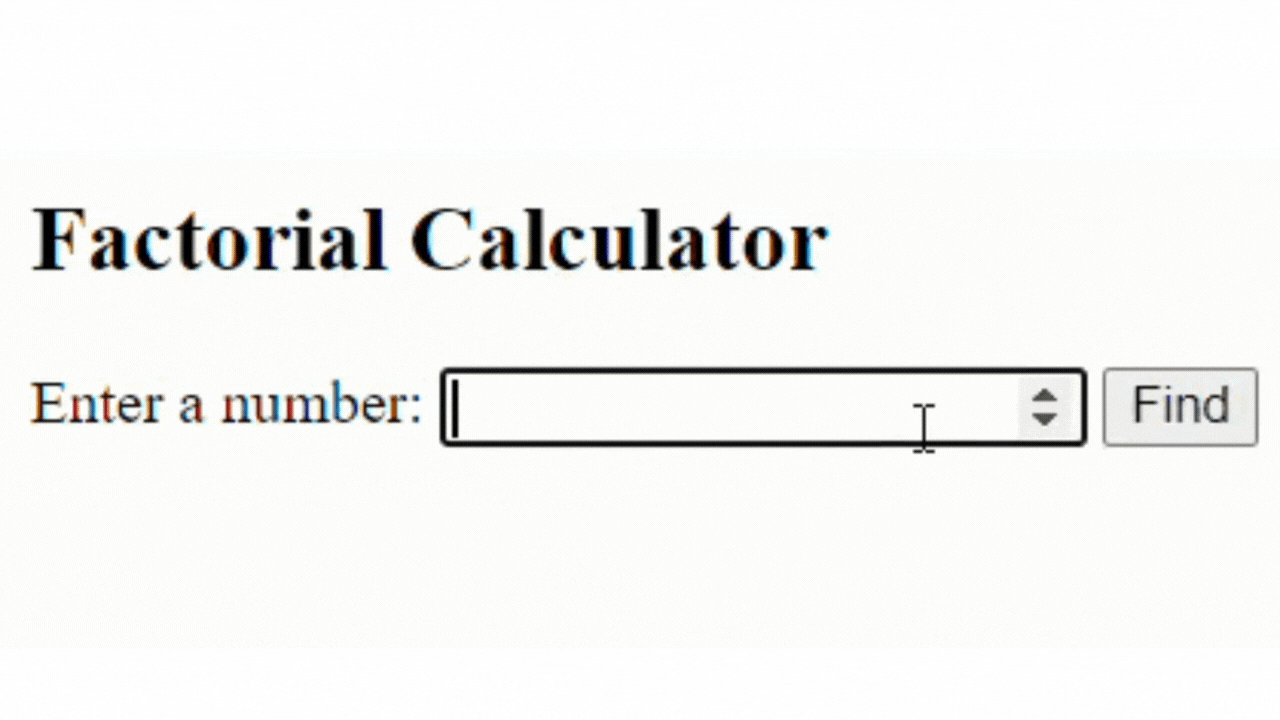# JavaScript Code to Find Factorial of a NumberHi developers, in this post you will learn how to Write a Program in JavaScript to find the factorial of a number in different ways.

Before that let's understand:

#### What is the Factorial of a Number?

The factorial of a number is the product of all the numbers from 1 to the given number.

Let's assume the number is 5 So the factorial of 5 will be:

1*2*3*4*5 = 120

Now, let's see what is the factorial of n numbers:

factorial of n(n!) = 1 * 2 * 3 * 4.....n

Now it's time to learn Different Ways of Finding the Factorial of a number in JavaScript:

### Factorial of a Number in JavaScript using for Loop

function factorial(number) {
if (number === 0 || number === 1) {
return 1;
}
else {
let result = 1;
for (let i = 2; i <= number; i++) {
result *= i;
}
return result;
}
}

console.log(factorial(5));

// Output: 120

### Find Factorial of a Number in HTML using JavaScript

<!DOCTYPE html>
<html>
<title>Finding Factorial of a Number using JavaScript </title>
<body>

<h2>Factorial Calculator</h2>
<form>
Enter a number:
<input type="number" id="inputValue">
<button type="button" onclick="FindFactorial()">Find</button>
</form>
<p id="outputArea"></p>

</body>
<script>
function FindFactorial() {
let number = parseInt(document.getElementById("inputValue").value);
let result = 1;

if (number === 0 || number === 1) {
result = 1;
}
else {
for (let i = 2; i <= number; i++) {
result *= i;
}
}

document.getElementById("outputArea").innerHTML = "Factorial of " + number + " is: " + result;
}
</script>
</html>

Output:### Factorial Program in JavaScript using while Loop

function factorial(number) {
if (number === 0 || number === 1) {
return 1;
}
else {
let result = 1;
let i = 2;
while (i <= number) {
result *= i;
i++;
}
return result;
}
}

console.log(factorial(4));

// Output: 120

### Factorial Program in JavaScript using Function

function factorial(number) {
if (number === 0 || number === 1) {
return 1;
}
else {
return number * factorial(number - 1);
}
}

console.log(factorial(5));

// Output: 120

Tags